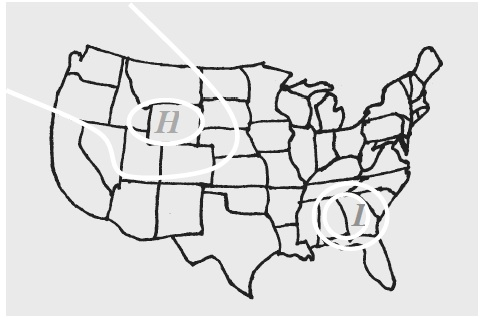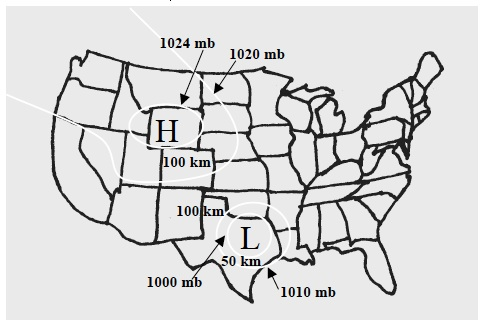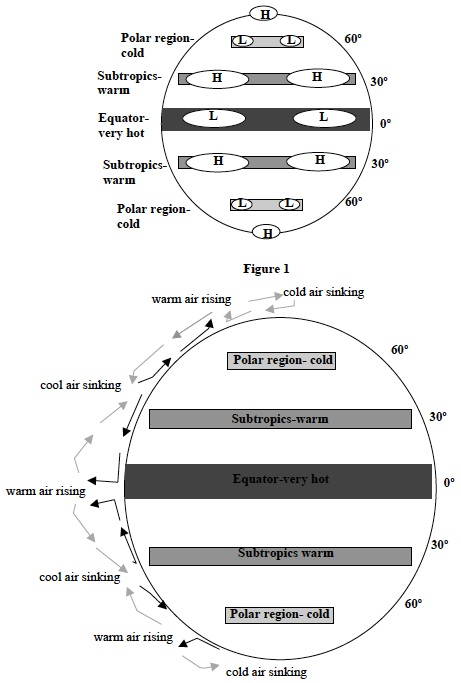# Investigation 2 – PostLab

### ANALYZE IT

Instruct students to complete the Analysis Questions in their SDRs then discuss them as a class. Use the suggested responses below to guide students’ answers.

• The weather map below shows areas of high and low pressure. Describe what is happening to the air at each of these locations.• In Investigation One you learned that cold air sinks and warm air rises. What type of pressure center would develop in an area in which a large amount of warm air is rising? Why?
• What type of pressure center would develop in an area in which a large amount of cold air is sinking? Why?

• In which direction did the aluminum foil pieces move when the high-pressure center was surrounded by areas of lower pressure?
• In which direction did the aluminum foil pieces move when the low-pressure center was surrounded by areas of higher pressure?
• Wind is the horizontal movement of air between areas of different pressure. In which direction does the wind blow? Explain your answer in terms of differences in pressure using data from your experiment.
• How did the strength of wind change as the distance between two areas of pressure increased?

• Calculate the PGF (pressure gradient force) for the winds produced in Trial 2.• How did the pressure gradient force a change as the distance between either the high or low-pressure center and other areas of pressure increase?

• The map below shows different isobars of pressure across the United States. Determine the pressure gradient force for the winds that will occur. Use the pressure gradient force to describe the strength and directions of the winds that will occur.• In which direction did the water in the barometer move when an area of high pressure was modeled?
• In which direction did the water in the barometer move when an area of low pressure was modeled?

• “The barometer is falling” is one phrase used to forecast changes in weather. What does this phrase indicate about the change in pressure that will occur over an area?
• Two barometers are shown below. What is the barometric pressure of each? Which shows a higher atmospheric pressure?### GET FOCUSED

Complete the Focus Questions in your SDR then discuss them as a class.

• What types of air movements cause areas of high and low pressure?
• Why do differences in pressure cause wind?
• How are differences in pressure measured?

### EXTEND IT

Figure 1 shows the temperature of different areas of the Earth. It also shows what meteorologists call semi-permanent high and low-pressure systems.

Figure 2 shows the movement of warm and cold air between the Earth and the atmosphere.• Why do you think that the semi-permanent high-pressure systems develop at 30 degrees and 90 degrees latitude? Why do you think semi-permanent low-pressure systems develop at 0 degrees and 60 degrees latitude?